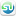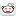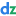## Generating Sequences

I was having a discussion with my son over breakfast about C++ and Python, and he asked me if C++ had anything equivalent to Python's `range()` function for generating a sequence of integers. I had to tell him that no, the C++ standard library didn't supply such a function, but there were algorithms for generating sequences (`std::generate` and `std::generate_n`) into an existing container, and you could write something that would provide a "virtual" container that would supply a sequence as you iterated over it with range-`for`.

He was happy with that, but I felt the urge to write such a container, and blog about it. There are existing implementations available (e.g. `boost::counting_range`), but hopefully people will find this instructive, if not useful.

### Iterating over a "virtual" container

Our initial goal is to be able write

``````for(int x: range(10))
std::cout<<x<<std::endl;``````

and have it print out the numbers 0 to 9. This requires that our new `range` function returns something we can use with range-`for` — something for which we can call `begin` and `end` to get iterators.

``````    class numeric_range{
public:
class iterator;
iterator begin();
iterator end();
};

numeric_range range(int count);``````

Our container is virtual, so we don't have real values we can refer to. This means our iterators must be input iterators — forward iterators need real objects to refer to. That's OK though; we're designing this for use with range-`for`, which only does one pass anyway.

#### Input Iterator requirements

All iterators have 5 associated types. They can either be provided as member types/`typedef`s, or by specializing `std::iterator_traits<>`. If you provide them as part of your iterator class, then the `std::iterator_traits<>` primary template works fine, so we'll do that. The types are:

##### `iterator_category`

The tag type for the iterator category: for input iterators it's `std::input_iterator_tag`.

##### `value_type`

This is the type of the element in the sequence. For an integer sequence, it would be `int`, but we'll want to allow sequences of other types, such as `unsigned`, `double`, or even a custom class.

##### `reference`

The result of `operator*` on an iterator. For forward iterators it has to be an lvalue reference, but for our input iterator it can be anything convertible to `value_type`. For simplicity, we'll make it the same as `value_type`.

##### `pointer`

The return type of `operator->` on an iterator. `value_type*` works for us, and is the simplest option.

##### `difference_type`

The type used to represent the difference between two iterators. For input iterators, this is irrelevant, as they provide a one-pass abstraction with no exposed size. We can therefore use `void`.

The types aren't the only requirements on input iterators: we also have to meet the requirements on valid expressions.

#### Input Iterator expression requirements

Input iterators can be valid or invalid. If an iterator is invalid (e.g. because it was invalidated by some operation), then doing anything to it except assigning to it or destroying it is undefined behaviour, so we don't have to worry about that. All requirements below apply only to valid iterators.

Valid iterators can either refer to an element of a sequence, in which case they are dereferencable, or they can be past-the-end iterators, which are not dereferencable.

##### Comparisons

For iterators `a` and `b`, `a==b` is only a valid expression if `a` and `b` refer to the same sequence. If they do refer to the same sequence, `a==b` is `true` if and only if both `a` and `b` are past-the-end iterators, or both `a` and `b` are dereferencable iterators that refer to the same element in the sequence.

`a!=b` returns `!(a==b)`, so is again only applicable if `a` and `b` are from the same sequence.

##### Incrementing

Only dereferencable iterators can be incremented.

If `a` is dereferencable, `++a` must move `a` to the next value in the sequence, or make `a` a past-the-end iterator. Incrementing an iterator `a` may invalidate all copies of `a`: input iteration is single-pass, so you cannot use iterators to an element after you've incremented any iterator that referenced that element. `++a` returns a reference to `a`.

`(void)a++` is equivalent to `(void)++a`. The return type is unspecified, and not relevant to the increment.

##### Dereferencing

For a dereferencable iterator `a`, `*a` must return the `reference` type for that iterator, which must be convertible to the `value_type`. Dereferencing the same iterator multiple times without modifying the iterator must return an equivalent value each time. If `a` and `b` are iterators such that `a==b` is `true`, `*a` and `*b` must be return equivalent values.

`a->m` must be equivalent to `(*a).m`.

`*a++` must return a value convertible to the `value_type` of our iterator, and is equivalent to calling following the function:

``````iterator::value_type increment_and_dereference(iterator& a) {
iterator::value_type temp(*a);
++a;
return temp;
}``````

This is why the return type for `a++` is unspecified: for some iterators it will need to be a special type that can generate the required value on dereferencing; for others it can be a reference to a real `value_type` object.

### Basic implementation

Our initial implementation can be really simple. We essentially just need a current value, and a final value. Our dereferencable iterators can then just hold a pointer to the range object, and past-the-end iterators can hold a null pointer. Iterators are thus equal if they are from the same range. Comparing invalidated iterators, or iterators from the wrong range is undefined behaviour, so that's OK.

``````class numeric_range{
int current;
int final;
public:
numeric_range(int final_):current(0),final(final_){}

iterator begin(){ return iterator(this); }
iterator end() { return iterator(nullptr); }

class iterator{
numeric_range* range;
public:
using value_type = T;
using reference = T;
using iterator_category=std::input_iterator_tag;
using pointer = T*;
using difference_type = void;

iterator(numeric_range* range_):range(range_){}

int operator*() const{ return range->current; }
int* operator->() const{ return &range->current;}

iterator& operator++(){ // preincrement
++range->current;
if(range->current==range->final)
range=nullptr;
return *this;
}

??? operator++(int); // postincrement

friend bool operator==(iterator const& lhs,iterator const& rhs){
return lhs.range==rhs.range;
}
friend bool operator!=(iterator const& lhs,iterator const& rhs){
return !(lhs==rhs);
}
};
};

numeric_range range(int max){
return numeric_range(max);
}``````

Note that I've left out the definition of the iterator postincrement operator. That's because it warrants a bit more discussion.

Remember the spec for `*a++`: equivalent to calling

``````iterator::value_type increment_and_dereference(iterator& a) {
iterator::value_type temp(*a);
++a;
return temp;
}``````

Since this is a combination of two operators, we can't do it directly: instead, our postincrement operator has to return a special type to hold the temporary value. Our postincrement operator thus looks like this:

``````class numeric_range::iterator{
class postinc_return{
int value;
public:
postinc_return(int value_): value(value_){}
int operator*(){ return value; }
};
public:
postinc_return operator++(int){
postinc_return temp(range->current);
++*this;
return temp;
}
};``````

That's enough for our initial requirement:

``````int main(){
for(int x: range(10)){
std::cout<<x<<",";
}
std::cout<<std::endl;
}``````

will now print 0 to 9.

### More complete implementation

The Python `range` function provides more options than just this, though: you can also specify start and end values, or start, end and step values, and the step can be negative. We might also like to handle alternative types, such as `unsigned` or `double`, and we need to ensure we handle things like empty ranges.

Alternative types is mostly easy: just make `numeric_range` a class template, and replace `int` with our new template parameter `T` everywhere.

Setting the initial and final values is also easy: just provide a new constructor that takes both the current and final values, rather than initializing the current value to 0.

Steps are a bit more tricky: if the `final` value is not `initial+n*step` for an integer `n`, then the direct comparison of `current==final` to check for the end of the sequence won't work, as it will always be `false`. We therefore need to check for `current>=final`, to account for overshoot. This then doesn't work for negative steps, so we need to allow for that too: if the step is negative, we check for `current<=final` instead.

### Final code

| Stumble It!| Submit to Reddit| Submit to DZone